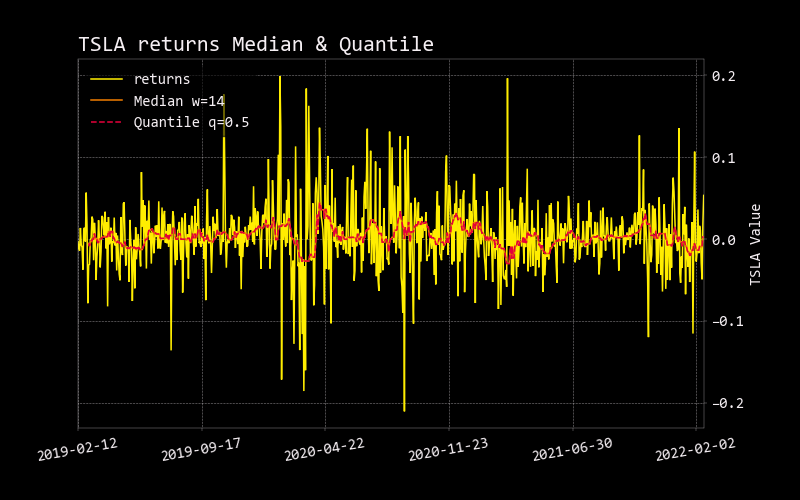# Quantile

``````usage: quantile [-l N_LENGTH] [-q F_QUANTILE] [--export {csv,json,xlsx}] [-h]
``````

In statistics and probability, quantiles are cut points dividing the range of a probability distribution into continuous intervals with equal probabilities, or dividing the observations in a sample in the same way.

The quantiles are values which divide the distribution such that there is a given proportion of observations below the quantile. For example, the median is a quantile. The median is the central value of the distribution, such that half the points are less than or equal to it and half are greater than or equal to it. By default, q is set at 0.5, which effectively is median. Change q to get the desired quantile (0 < q < 1).

See the Wiki page on this topic for further explanation: https://en.wikipedia.org/wiki/Quantile

``````optional arguments:
-l N_LENGTH, --length N_LENGTH
length (default: 14)
-q F_QUANTILE, --quantile F_QUANTILE
quantile (default: 0.5)
--export {csv,json,xlsx}
Export dataframe data to csv,json,xlsx file (default: )
-h, --help            show this help message (default: False)
``````